# Oval

Updated on
Covid-19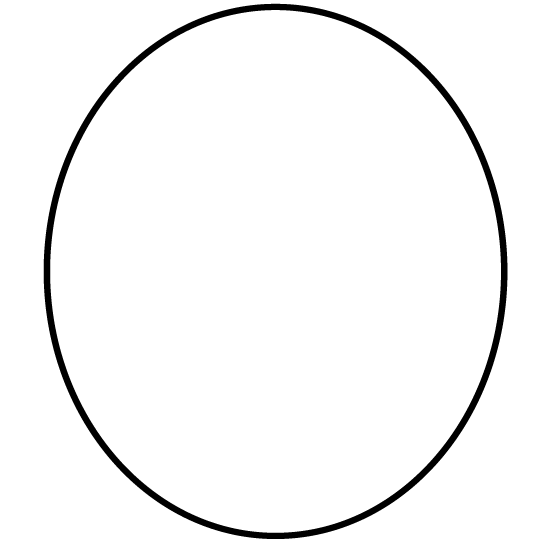## Oval 94 diskont full album

An oval (from Latin ovum, "egg") is a closed curve in a plane which "loosely" resembles the outline of an egg. The term is not very specific, but in some areas (projective geometry, technical drawing, etc.) it is given a more precise definition, which may include either one or two axes of symmetry. In common English, the term is used in a broader sense: any shape which reminds one of an egg. The three-dimensional version of an oval is called an ovoid.

## Contents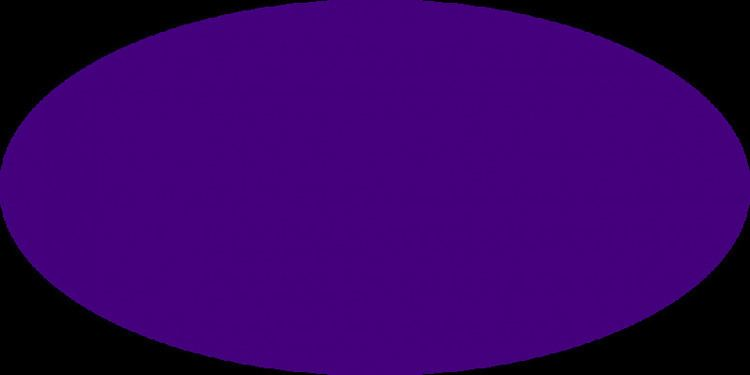## Oval in geometry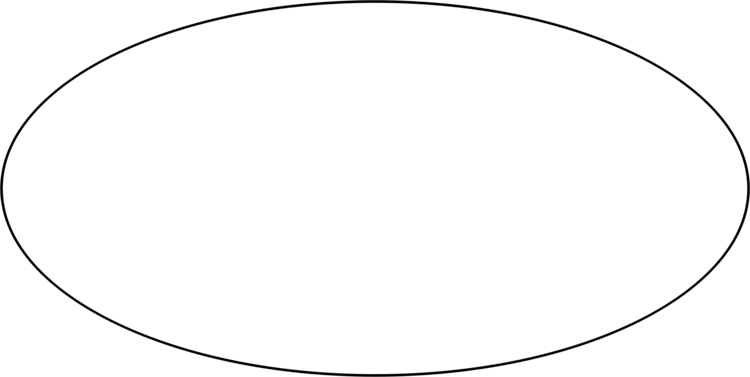The term oval when used to describe curves in geometry is not well-defined, except in the context of projective geometry. Many distinct curves are commonly called ovals or are said to have an "oval shape". Generally, to be called an oval, a plane curve should resemble the outline of an egg or an ellipse. In particular, these are common traits of ovals: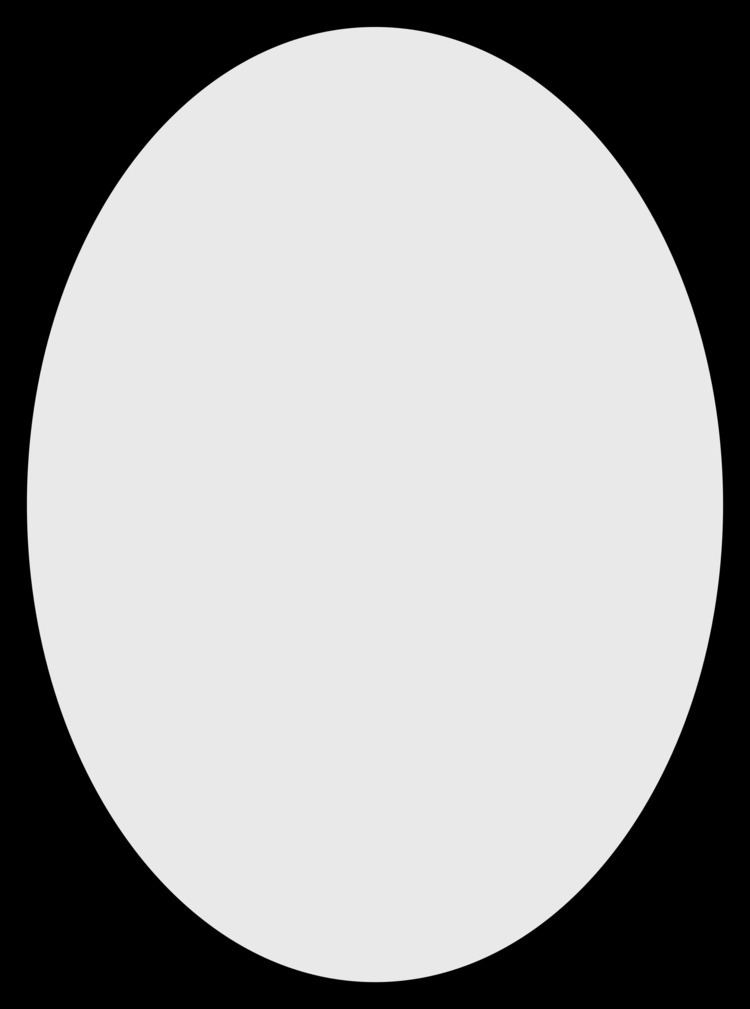• they are differentiable (smooth-looking), simple (not self-intersecting), convex, closed, plane curves;
• their shape does not depart much from that of an ellipse, and
• there is at least one axis of symmetry.
• Here are examples of ovals described elsewhere:

• Cassini ovals
• elliptic curves
• Moss's Egg
• superellipse
• Cartesian oval

•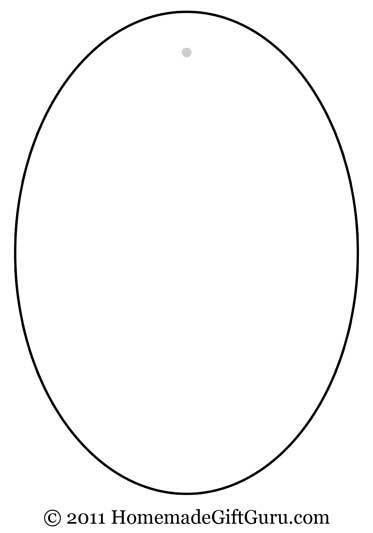An ovoid is the 2-dimensional surface generated by rotating an oval curve about one of its axes of symmetry. The adjectives ovoidal and ovate mean having the characteristic of being an ovoid, and are often used as synonyms for "egg-shaped".

## Projective geometry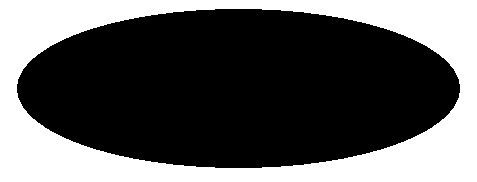In the theory of projective planes, an oval is a set of n + 1 points in a projective plane of order n, with no three on a common line (no three points are collinear).

An ovoid in the finite projective geometry PG(3,q) is a set of q2 + 1 points such that no three points are collinear. At each point of an ovoid all the tangent lines to the ovoid lie in a single plane.

## Egg shape

The shape of an egg is approximated by "long" half of a prolate spheroid, joined to a "short" half of a roughly spherical ellipsoid, or even a slightly oblate spheroid. These are joined at the equator and sharing a principal axis of rotational symmetry, as illustrated above. Although the term egg-shaped usually implies a lack of reflection symmetry across the equatorial plane, it may also refer to true prolate ellipsoids. It can also be used to describe the 2-dimensional figure that, if revolved around its major axis, produces the 3-dimensional surface.

## Technical drawing

In technical drawing, an oval is a figure constructed from two pairs of arcs, with two different radii (see image on the right). The arcs are joined at a point in which lines tangential to both joining arcs lie on the same line, thus making the joint smooth. Any point of an oval belongs to an arc with a constant radius (shorter or longer), but in an ellipse, the radius is continuously changing.

## In common speech

In common speech, "oval" means a shape rather like an egg or an ellipse, which may be two-dimensional or three-dimensional. It also often refers to a figure that resembles two semicircles joined by a rectangle, like a cricket infield or athletics track. However, this is more correctly called a stadium or archaically, an oblong. Sometimes, it can even refer to any rectangle with rounded corners.

The shape lends its name to many well-known places.

Oval Wikipedia

Topics